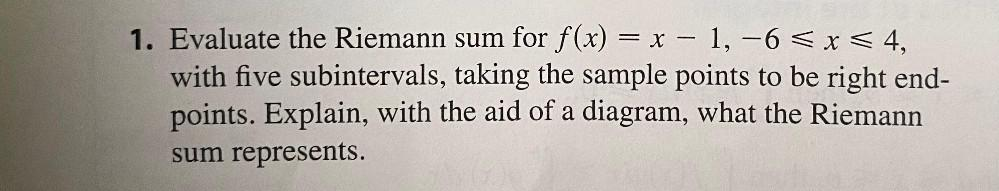Question:

# = 1. Evaluate the Riemann sum for f(x) = x – 1, -6 < x < 4, with five subintervals, taking the sample points to be right end- po= 1. Evaluate the Riemann sum for f(x) = x – 1, -6 < x < 4, with five subintervals, taking the sample points to be right end- points. Explain, with the aid of a diagram, what the Riemann sum represents.